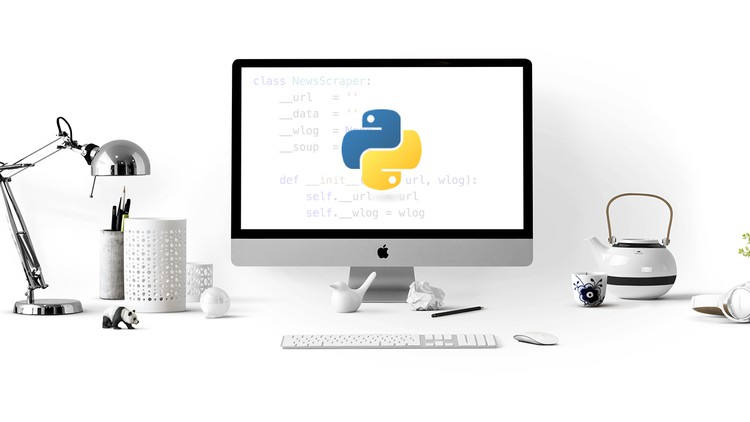## This course is a depth introduction to both fundamental python programming concepts and the Python programming language.

What you will learn

Getting Started, Python From Command line, Values and Data Types, Operators and Operands, Function Calls

Write scripts for general productivity tasks Read and comprehend Python code Gain knowledge in regard to general programming concepts

Use variables to store, retrieve and calculate information

Data Types, Type Conversion, Functions, Statements and Expressions

Mathematical Function, Random Number Function, Trigonometric Functions, Trigonometric Inverse Functions

CGI Programming, Web Server Configuration

Description

This course is a depth introduction to fundamental python programming concepts.

Learn Python From Beginner To Advanced Level

• I’ve created thorough, extensive, but easy to follow content which you’ll easily understand and absorb.The course starts with the basics, including Python fundamentals, programming, and user interaction.The curriculum is going to be very hands-on as we walk you from start to finish becoming a professional Python developer. We will start from the very beginning by teaching you Python basics and programming fundamentals, and then going into advanced topics and different career fields in Python so you can get real-life practice and be ready for the real world.
• The topics covered in this course are:* Beginner to Expert Python contents:Array implementationFile methodsKeywords and IdentifiersPython Tuples

Python Basics

Python Fundamentals

Mathematical Functions

Data Structures

Object-Oriented Programming with Python

Functional Programming with Python

Lambdas

Decorators

Generators

Testing in Python

Debugging

Error Handling

Regular Expressions

Comprehensions

Modules

• See you inside the course!

## English

Language

Content

Python For Beginners Course In-Depth

Tutorial 1-Python From Command Line

Tutorial 2-Python Script From Command Line

Tutorial 3-Python Command Line Options

Tutorial 4-Python Language Overview

Tutorial 5-IDLE Interactive Shell

Tutorial 6-Python Basic Syntax

Tutorial 7-Python Keywords

Tutorial 8-python Lines And Indentation

Tutorial 9-Python Identifiers

Tutorial 10-Python Quotations

Tutorial 11-Python Multiline Statements

Tutorial 12-Python Command line Argument

Tutorial 13-Python Standard Data Types

Tutorial 14-Python Numbers

Tutorial 15-Python Mathematical Functions

Tutorial 16-Python Random Number Functions

Tutorial 17-Python Trignometric Functions

Tutorial 18-Python Mathematical Constants

Tutorial 19-Trigonometric Inverse Functions

Tutorial 20-Trigonometric Misc Functions

Tutorial 21-Python Operators

Tutorial 22-Python Arithmetic Operators

Subscribe to latest coupons on our Telegram channel.

Tutorial 23-Python Bitwise Operators

Tutorial 24-Python Logical Operators

Tutorial 25-Python Membership Operators

Tutorial 26-Python Identity Operators

Tutorial 27-Python Operator Precedence

Tutorial 28-Python Strings

Tutorial 29-String Basic Operators

Tutorial 30-String Formatting Operators

Tutorial 31-Python Triple Quotes

Tutorial 32-String Encoding Functions

Tutorial 33-Python List

Tutorial 34-Access Update & Delete List

Tutorial 35-Basic List Operations

Tutorial 36-Indexing, Slicing & Matrixes

Tutorial 37- Python List Methods

Tutorial 38-Built in List Functions

Tutorial 39-Python Decision Making

Tutorial 40-Python If Statement

Tutorial 41-Python if else statement

Tutorial 42-Python if elif else statement

Tutorial 43-Python single statement suites

Tutorial 44-Python Loops

Tutorial 45-Python While loop

Tutorial 46-python Infinite While Loop

Tutorial 47-while loop else statement

Tutorial 48-While Loop Single Statement Suites.

Tutorial 49-Python for loop

Tutorial 50-For Loop Using rang() Function

Tutorial 51-for loop iterating by sequence index.

Tutorial 52-Python Nested loops

Tutorial 53-Loop control statement